## 02 November 2010

### How to Determine MOSFET Type from Circuit Symbol

I have noticed that my book does not present an easy way to quickly tell the difference between a) different types of MOSFETs and b) different MOSFET circuit configurations.  So let's work it out.

First, let's note that there are two types of MOSFETs.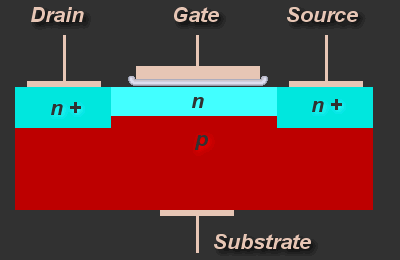Fig 1. N-channel MOSFET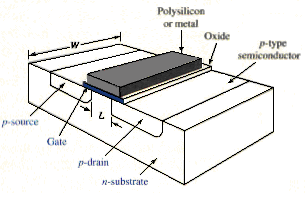Fig 2. P-channel MOSFET

Okay, this is the easy part.  We know that when we have a p-type substrate with n-type source and drain regions, we get an n-channel MOSFET.  The opposite is true for a p-channel MOSFET.  Now, how about the circuit symbols?

So, we have a gate, source, drain, and body connection (the body is normally shorted to either the source or the drain, most commonly the source).  Then we have that little arrow...  What's up with that?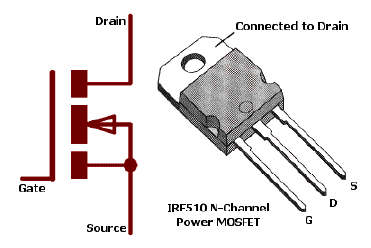Fig 3.  MOSFET (body shorted to source)

In figure 3, the arrow is pointing toward the gate.

Here are some possibilities...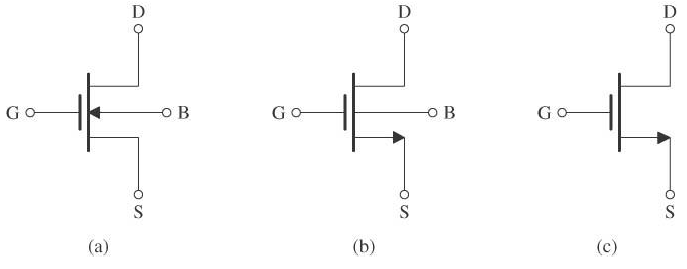Fig 4.  Three circuit symbols for an n-channel MOSFET

(a) is the circuit symbol for an n-channel enhancement-type MOSFET
(b) is the circuit symbol for an n-channel MOSFET (with body pin shown)
(c) is the circuit symbol for an n-channel MOSFET (with out the body pin shown)

Differences?

If the body pin is shorted to the source or if the body pin does not affect the operation of the MOSFET, it is left out.  But wait, in (a), the arrow points toward the gate and in the other two, it points away from the gate!

I have to dig back into what I learned before and recall.  We define that polarity is positive if current flows INTO the positive terminal and out of the negative terminal.  I therefore make my first assumption.  The arrow indicates the polarity of the device, with respect to the gate.  This must mean that when the device is on, there is a positive gate voltage, creating an n-channel!  Woohoo.

I will say that, almost all of the simple circuits I have seen use (c) as the symbol for the n-channel MOSFET.  Just to clarify, the circuit in (a) does not show that the body and source pins are usually shorted together.  When the body pin and the source pin are shorted together, the arrow can change direction if placed over the source pin.  Otherwise, if the body pin is shorted to the source pin, (a) and (c) are equivalent.

So, there we have it.  If the arrow is pointing away from the gate, that means that the device is on when there is a positive gate voltage, creating an n-channel.  If the arrow is pointing toward the gate, that means that the device is on when there is a negative gate voltage, creating a p-channel.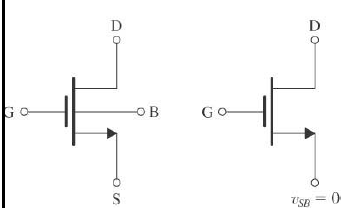Fig 5.  N-channel MOSFET (notice V_sb = 0 implies B shorted to S)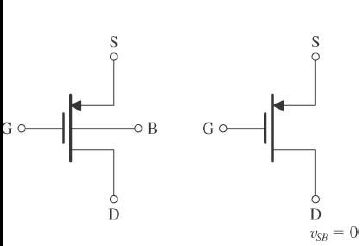Fig 6.  P-channel MOSFET (same note as above)

Keep in mind that I spoke very loosely about the device being on.  When I said that, I assume that the appropriate thresholds have been met (i.e. V_gs > V_t for an n-channel).

I hope this helps.  It helped me!

[all images retrieved via Google images or Microelectronic Circuits, Sedra/Smith, 6th]